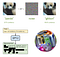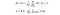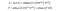Research on Adversarial Attack against Graph Neural Network.

Deep Learning Networks are prone to be messed with “Adversarial Attacks.” Now this problem has come to haunt Graph Neural Networks. In this post, 2 papers and a tutorial are summarized.

1. Overview

[In short]
An Adversarial Attack is a process of creating invisible patterns that cause neural networks to fail. (This describes a norm-bounded test-time attack).Top: famous panda attacked by adversarial noise. Bottom Left: GPT-2 leaking intel (this post). Bottom Right: an Adversarial Patch from this paper.

An Adversarial Attack is any method that exploits the weakness of the targeted system to cause it to work in a way that is unintended or undesirable for the original creator of the system. Examples:
— A neural network trained to classify cat and dog images → add imperceptible “adversarial noise” to cause misclassification.
— A neural network trained to detect cars on streets → print an “adversarial patch” of color with a seemingly random color and fool the system into detecting non-existing cars.

— Modify and “poison” the training dataset and cause the neural network to misclassify or misbehave in targeted scenarios.
— Extract information of training data from the model. For example, GPT-2 leaked a particular individual's contact information.

The four scenarios can be named norm-bounded test-time attack, unbounded attack, poisoning attack and inference attack.

Notice in the first two scenario examples, the modifications are limited in amplitude (imperceptible) or localized (a patch and not the entire region). This is because, in current academic research of adversarial attacks, unlimited modifications are considered as out-of-scope of the deep learning system. However, more extreme cases may also need to be considered in practical use.

The first example describes the “norm-bounded adversarial attack,” which has a standard mathematical definition:

which says: create an adversarial example x’ bounded by a small epsilon (norm-p) distance from a legitimate example x, but causes the neural network function f to respond differently.

The overall scheme is that deep (intelligent?) learning systems are expected to behave by human standards — if indistinguishable noise do not affect our perception, we suppose NNs should also not be affected. If random noise does not resemble anything to us, we expect NNs to not pick up on random colors and detect object where there isn’t.

Attack on GNN

“Human standards” for graph data can be less intuitive. In the following works, adversarial attack objectives are derived from the norm-bounded attack. With minimum alteration on graphs, the adversary aims to mess up GNN’s ability to conduct algorithms on graphs (graph classification, node classification, link prediction,… ), while being unnoticeable.

2. outlines

Adversarial Attacks on Neural Networks for Graph Data, Zügner et al., KDD 2018.

What is the problem?

The internet is full of adversaries, and false data are easy to inject.

The above situation brings up the question: are Graph Neural Networks for node classification robust against adversarial attack? As this work was the first to probe the question, the authors analyzed the potentials and challenges for the adversary:

[potential of attack]

• There are more possibilities of modification on graphs because both node features and the graph structure can be modified.
• Since GNN propagates information, modification on some nodes (attacked) may influence others (targets).

[challenges of attack]

• Graph features are discrete, gradient descent optimizations would fail.
• How do we define an “unnoticeable change” to a graph?
• Node classification questions train and test on the same (large) graph, i.e., particular nodes are left out in training and in testing. This causes adversarial attacks to become like the (more difficult) poisoning attacks.

The work focused on GCN with a single layer. They assume that the adversary can know the full graph but can only modify parts of the graph.

The Attack model

Graphs include nodes and edges G = (V, E), which can also be denoted by the connectivity of nodes and the node features G = (A, X).
It can be modified on A for structure attacks or on X for feature attacks.
The modification is limited to a subset of nodes W ⊂ V, either an edge connected to 1 or 2 of the nodes in W or the feature of a node in W.
The work denotes influencer attack if the target node v_0 is not in W and direct attack if the target node is in W.

The goal can be written in terms of log difference in classification confidences for the target node.

Unnoticeable change definitions

1. The number of changes is limited by a budget ∆:

However, this is a naive restriction because compared to the adversarial attack on images: (i) such changes are still discrete and not infinitesimal (ii) for large graphs, visual inspection is not realistic, while statistic information is utilized. In particular, degree distribution is the most prominent characteristic of the graph structure. Thus the second restriction is proposed:

2. Statistical test for the node degree distribution (structure statistic)

Assuming degree distribution follows power-law distribution: p(x) ∝ x^[−α] (True in real networks). We can estimate the scaling parameter α for samples D (nodes with degree > d_min on) the original graph G(0) and the modified graph G’ as well as the combined graph (comb, sample from both graphs).

From the estimation, log-likelihood can be calculated. The authors form 2 hypotheses (H_0 and H_1) and follow the likelihood ratio test to obtain the final test statistics. The numerical value is found from χ^2 distribution table (with 1 degree of freedom) and for p-value = 0.95.

3. Statistical test for node feature statistics.

While distribution statistics can also be applied to features, the authors argue that correlation/co-occurrence is much more important for features.

The authors consider the co-occurrence graph C = (F, E) of features from the original graph. In C, each feature is a node, and each edge denotes co-occurrence (on a node feature in G). (The nodes are assumed to have binary values for each feature). The authors argue that adding a particular feature i to a node v is unnoticeable if it already cooccurs with other features of node v, i.e., a random walker on C starting from the features of node u (S_u) has a high probability of reaching feature i with a single step.S_u is the set of all features u has, d_j is the degree of feature j on C. σ is 1/2 maximum achievable probability.

How is the problem solved?

Surrogate linear activation model:Target GCN model function and the simplified surrogate. Z_vc is the probability of node v to be classified as c.

Build surrogate loss and score for structure or feature modification actions on top of surrogate loss.

Fast computation of candidates —
structure: degree is altered additively, can calculate the incremental change to test statistics.
feature: candidate can be precomputed since the modification aims to preserve co-occurrence.

Fast computing the scores —
structure: only A changes, so only 2 hops from the proposed change needs to be addressed.
feature: since the structure is not changed, simply calculate the gradient and pick the one that occurs at the available direction (0 to 1 or 1 to 0, not 0 to negative)

Time complexity — for iteration budget ∆, each attack nodes W, check N edge✕2 hops, plus D features…… O(∆|W|(N✕hops + D))

Experimental Results

• direct attack easier, but influence attack is still possible
• test statistics change is noticeable if not constrained
feature (word choice) is also noticeable
• run time scales linearly
• poisoning attack also works
• only seeing 10% of the whole graph still works

Adversarial Examples on Graph Data: Deep Insights into Attack and Defense, Wu et al., IJCAI 2019.

Starting from a reference x’ (e.g. all-black image), a path integral is calculated on the gradient terrain.

For graphs, to calculate a certain edge change:

• if A_ij = 1, calculate removing edges, vice versa
• if removing, path integral from all 0 matrix => fraction connection (otherwise from all 1 matrix).
• is probably calculating the gradient of perturbing the (fractional edge strength of) edge_ij.

From the following observations, the author explains why adversarial retraining as a defense would work, and also propose to “clean” the graph data as an even simpler defense

• edge perturbation > feature modification
• add edge > remove edge
• less neighbor easier to attack
• attack tends to connect target node to dissimilar (feature) node
• To defend:
1) retraining, or
2)simply clean the dataset and remove the dissimilar connections.

Deep Graph Learning: Foundations, Advances and Applications, Rong et al., KDD 2020 tutorial.

(Section: Robustness of GNN, on presentation slide pages 126–169, youtube video recording 1:04:55 — 1:36:50)

outline

Robust GAN 1:04:55 start, introduction to adversarial attack, graph example (node classification → web identity),

1:08:50 image vs graph
1:10:10 perturbation types for graph,

Attacks
1:16:44 Nettack: surrogate + more constraint (statistics)
1:19:40 GF-attack: focus on the feature
1:22:00 ReWatt: focus on rewire (add + minus edge), with reinforcement learning

Defenses